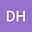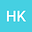Approach to the construction of the spaces $S{D^p}[\R^{\infty}]$ for $1 \leq p \leq \infty$
••The objective of this paper is to construct an extension of the class of Jones distribution Banach spaces $SD^p[\R^n], 1\le p\le \infty,$ which appeared in the book by Gill and Zachary \cite{TG} to $S{D^p}[\R^{\infty}]$ for $1\leq p \leq \infty.$ These spaces are separable Banach spaces, which contain the Schwartz distributions as continuous dense embedding. These spaces provide a Banach space structure for Henstock-Kurzweil integrable functions that is similar to the Lebesgue spaces for Lebesgue integrable functions.